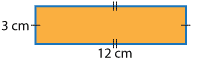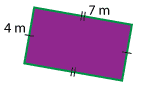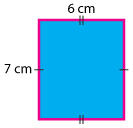#### Question 2

Calculate the area of these rectangles by filling in the boxes.

 aArea Formula of area= × widthFormula of area= 12 × Total of area= cm²
 bArea = length × widthFormula of area= 7 × Total of area= m²
 cArea Total area= what is the unit? ²